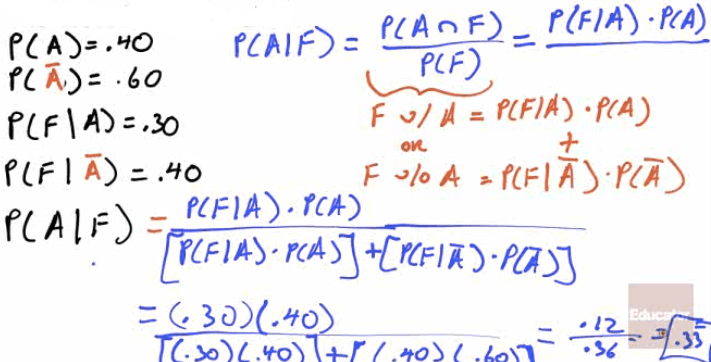# Probability Model

• A mathematical description of a random phenomenon.

• The Probability Model consists of

• Sample Space (S) = the set of all possible outcomes

• Event within the sample space = an outcome or set of outcomes in S

• Probabilities associated with each event# Disjoint Events (aka Mutually Exclusive)

• Disjoint / mutually exclusive example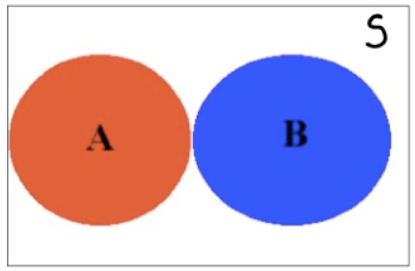• Not disjoint / not mutually exclusive example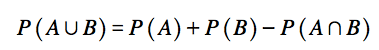# Independence vs. Dependence

• A conditional probability is the probability of some event occurring, given that some other event has already occurred. The conditional probability of event X occurring, given that some other event Y has already occurred, is written as P(X|Y).

• For example, P(M|N) would be the probability of the occurrence of event M given that event N has already occurred. It would be read as “the probability of M, given N.”

• As stated earlier, two events are considered independent if the occurrence of one of the events does not change the probability of the other event from what it would have been had the first event not occurred. Thus, two events, X and Y, are independent if P(X|Y) = P(X) or P(Y|X) = P(Y)

• Actually, these two conditional relationships are related. If one is true, the other must be true. If one is false, the other must be false.

• If P(X|Y) = P(X), then P(Y|X) = P(Y), and the events are independent.

• If P(X|Y) ≠ P(X), then P(Y|X) ≠ P(Y), and the events are dependent.

# Probability Rules

• Notation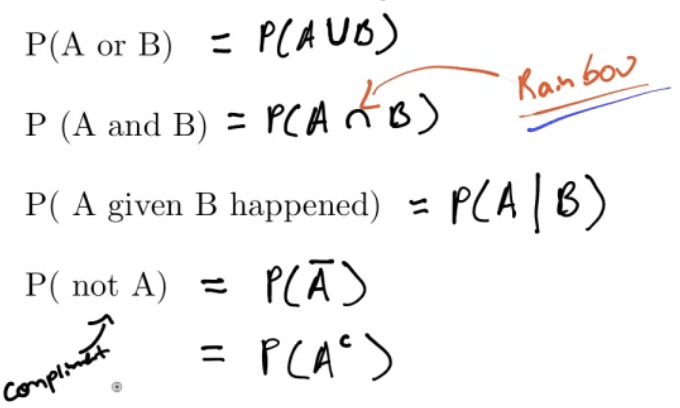• Rules# Overview# Bayes Rule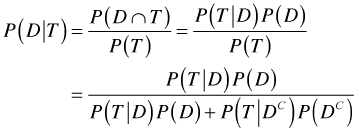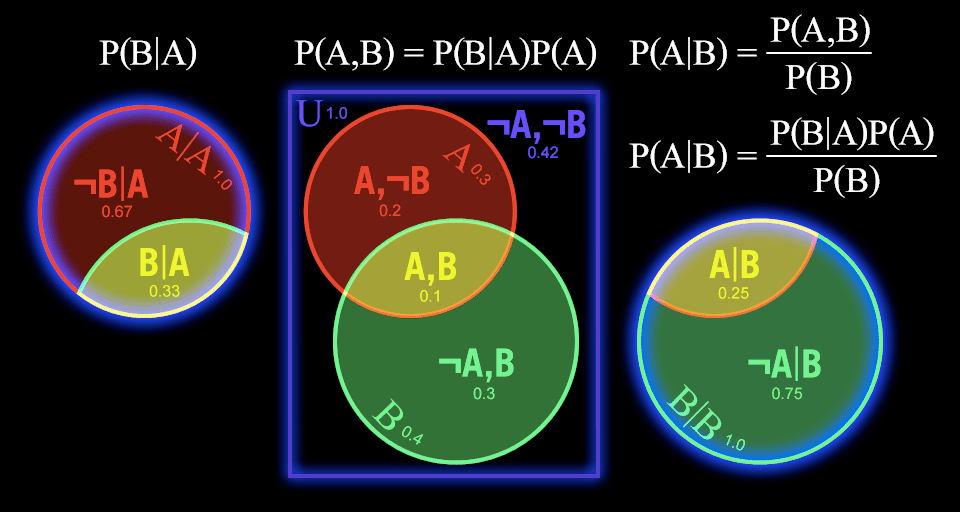# Simulations

• Imitating a real world process

1. Describe the possible outcomes

2. Determine the probability of each outcome

3. Link each outcome to one or more random numbers

4. Choose a random number for each "trial"

5. Based on the random number, note the "simulated" outcome

6. Repeat step 4 and 5 for each "simulation"

# Practice Questions

• A basketball player shoots 8 free throws during a game. We are interested in the probability that she makes 4.

• Describe the probability model in this case• Let A be the event that a victim of violent death was a woman and B the event that the death was a suicide. The proportion of suicides among violent deaths of woman is expressed in probability notation as

1. 0.5

2. 0.126

3. P(A and B)

4. P(A|B)

5. P(B|A)

• Suppose that for a group of consumers, the probability of eating pretzels is 0.65 and that the probability of drinking Coke is 0.75. The probability of eating pretzels and drinking Coke is 0.45. Determine if those two events are independent.

• P(P)=0.65

• P(C)=0.75

• P(P and C)=0.45

• P(P and C)≠P(P)*P(C)

• So P and C are not independent

• What is the probability that the students is either a female of at least 35 years old?• Generally speaking, 1% of college football players use steroids, but your friend on the football team has tested positive for steroid use. The blood test the league uses correctly comes up positive for 95% of steroid users, but mistakenly comes up positive for 1% of non-users. Given that your friend tested positive, how likely is it that he has been using steroids?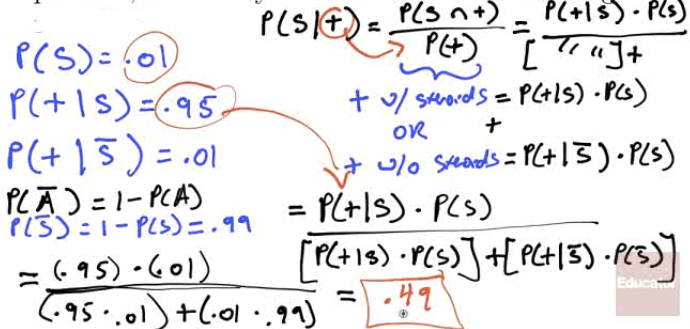• Of all the soda drinkers in a particular district, 40% prefer brand A and 60% prefer brand B. Of those drinkers who prefer brand A, 30% are females, and of those who prefer brand B, 40% are female. What is the probability that a randomly selected soda drinker prefers brand A, given that the person selected is a female